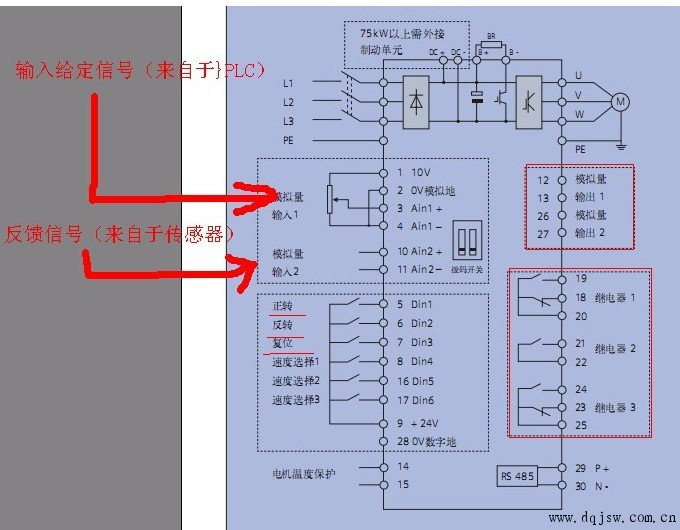# 西门子440变频器基本参数设置为了解决用户可能碰到关于"西门子440变频器基本参数设置"相关的问题，突袭网经过收集整理为用户提供相关的解决办法，请注意，解决办法仅供参考，不代表本网同意其意见,如有任何问题请与本网联系。"西门子440变频器基本参数设置"相关的详细问题如下:西门子440变频器基本参数设置
===========突袭网收集的解决方案如下===========

P0100=0
P0205=0
P0300=1
P0304=400
P0305=3.7
P0307=1.5
P0310=50
P0311=1425
P0700=1 BOP面板手动操作方式
P1000=1 BOP面板手动操作方式
P1080=0
P1082=50
P1120=10
P1121=10
P1135=5
P1300=20
P3900=1 变频器显示BUSY大约10秒后，完成快速调试。

P0756=2
P0757=4
P0758=0
P0759=20
P0760=100 %对应50Hz，80%对应40Hz，70%对应35Hz。
P0761=4.00 避免变频器由于4-20mA控制信号的飘移，低于4mA时，造成变频器反转，对低于4mA的控制信号，变频器均认为是4mA，且不会反转。

P0700=2 外部自动控制方式
P1000=2 外部自动控制方式

1,选择控制方式,面板或外部端子;
2,选择最低和最高工作频率;
3,选择是否反转禁止;
4,,选择过流电流;
5,选择加减速方式;
6,选择断电重启模式;

### 为您准备的相关内容:

• 西门子变频器440参数组设置步骤
• 西门子MM440变频器参数设置问题
• 西门子440变频器参数的设定。
• 怎样设置西门子变频器440的参数，要求电流输出
• 西门子440变频器参数如何调试？
• 西门子440变频器,如何调整参数,可以正反转?
• 西门子变频器参数设置方法介绍
• 西门子440变频器所有参数为什么不能改
• >>> 温馨提示:您还可以点击下面分页查看更多相关内容 <<<

## 热门

Copyright ? 2012-2016 tuxi.com.cn 版权所有 京ICP备10044368号 关于我们 | 广告服务 | 诚聘英才 | 联系我们 | 友情链接 | 免责申明 | 懂视